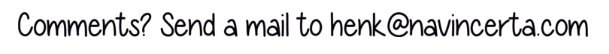# An innovation for decision tree probabilitiesThe attraction of a decision tree is that it brings together conceptual thinking about a decision problem and the quantitative evaluation of it. For the latter purpose it may be inferior to other methodologies, for example Monte Carlo simulation or analytical?approaches, however the combination of visualization and quantitative auditability for the benefit of decision makers is very powerful. It allows them to appreciate the decisions involved, their sequencing and the extent of the associated quantified uncertainties, in a transparent fashion.

Whilst the structure of such a tree needs to adequately represent the decision problem, which is an art in itself (not too complicated, focus on the key issues), the values and probabilities assigned to the branches of the tree, of course, need to be credible. Depending on the type of uncertainty that is depicted by a particular uncertainty node, the probabilities of the branches can come from a variety of sources. If the possible outcomes of the uncertainty are distinct different hypotheses, then judgmental probability assessments are required. Sometimes, however, the outcomes identified are just discretizations of an existing continuous probability distribution that describes the uncertain quantity. This article zooms in on this discretization topic.

To represent such a continuous variable in a decision tree usually three discrete values (or: cases) are selected to depict the range of uncertainty. These cases need to be assigned probabilities for the tree to be evaluated. A widely used approximation is assigning a probability of 0.25 to the low and the high cases, and 0.50 to the mid case. Another approach is the so called Swanson rule, which uses 0.3 for the low and high, and 0.4 for the mid case. In a paper published in 2011 in the SPE Journal of Economics and Management (see reference below) a comprehensive review of the various methods has been presented. The study has analyzed the inaccuracies in the above mentioned rules of thumb and presents an alternative based on the Gaussian quadrature approach. The result is a set of distribution-specific weighting factors that can be applied provided the discretization values are taken at specified percentile values. The advantage is that the discretized values with their probabilities are precise representations of the continuous distributions, as multiple distribution moments*) are matched.

In this article we would like to present a (we believe) new method for discretization that applies to any distribution and for which the discrete values, for example to be used in the decision tree, do not need to be fixed at certain percentile values. In fact, it is not even needed to know the percentile values of the discrete cases.

What is required is that one knows the mean, standard deviation and skewness of the continuous distribution. In addition, one needs to have chosen values for two of the three discrete cases; the third value will follow from a simple calculation procedure, in addition to the probabilities or weights. This is explained in the following 2 minute video clip.

For further details and background, including the mathematical derivation, one is referred to our free knowledge base where an article on the topic can be found.

As apparent from the video clip, the calculation is easily coded in a simple spreadsheet. In some cases one may wish to ignore the requirement to match the skewness (for example if the distribution is near-symmetric); if so, the goal seek element can be omitted. Although theoretically the discrete values can be chosen at random (as the weights will be adjusted so the moments will match), it is advisable to choose the values such that the weights assume ‘reasonable and intuitive’ numbers (> 0 and < 1).

In the development of this method I have been assisted by my business partner Thijs Koeling who came up with the clever idea of adding the goal seek element for matching the skewness. We believe that the aggregate of the formulas and calculation procedure constitute an exciting new tool in the domain of decision, risk and uncertainty analysis.

Yet, we do not necessarily advise against the use of the approximation methods such as Swanson’s rule. Their accuracy is adequate for common symmetric and slightly skewed distributions, whilst using the classic set of P90, P50 and P10 percentiles.

The advantage of having this new method available in the toolkit of the analyst is the flexibility to work with any discrete values as well as its applicability for any type of continuous distribution to be discretized.

In addition, one is assured that the first three moments are correctly represented by the discrete distribution and thus in the decision tree.

Reference: Discretization, Simulation, and Swanson?s (Inaccurate) Mean, J. Eric Bickel, SPE, and Larry W. Lake, SPE, The University of Texas at Austin, John Lehman, Strategic Decisions Group, SPE?Economics and Management, 2011.

*) A moment, as used in this context, is a quantitative measure characterizing a probability distribution. The first moment is the mean or expectation. The second (central) moment is the variance (the square of the standard deviation); this is a measure of the width of the distribution. The third (central) moment is a measure of the skewness of the distribution.